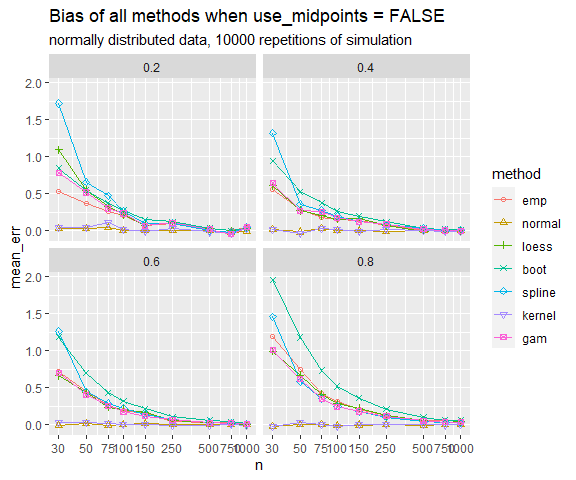# An introduction to cutpointr

#### 2022-04-13

cutpointr is an R package for tidy calculation of “optimal” cutpoints. It supports several methods for calculating cutpoints and includes several metrics that can be maximized or minimized by selecting a cutpoint. Some of these methods are designed to be more robust than the simple empirical optimization of a metric. Additionally, cutpointr can automatically bootstrap the variability of the optimal cutpoints and return out-of-bag estimates of various performance metrics.

## Installation

You can install cutpointr from CRAN using the menu in RStudio or simply:

install.packages("cutpointr")

## Example

For example, the optimal cutpoint for the included data set is 2 when maximizing the sum of sensitivity and specificity.

library(cutpointr)
data(suicide)
head(suicide)
##   age gender dsi suicide
## 1  29 female   1      no
## 2  26   male   0      no
## 3  26 female   0      no
## 4  27 female   0      no
## 5  28 female   0      no
## 6  53   male   2      no
cp <- cutpointr(suicide, dsi, suicide,
method = maximize_metric, metric = sum_sens_spec)
## Assuming the positive class is yes
## Assuming the positive class has higher x values
summary(cp)
## Method: maximize_metric
## Predictor: dsi
## Outcome: suicide
## Direction: >=
##
##     AUC   n n_pos n_neg
##  0.9238 532    36   496
##
##  optimal_cutpoint sum_sens_spec    acc sensitivity specificity tp fn fp  tn
##                 2        1.7518 0.8647      0.8889      0.8629 32  4 68 428
##
## Predictor summary:
##     Data Min.   5% 1st Qu. Median      Mean 3rd Qu.  95% Max.       SD NAs
##  Overall    0 0.00       0      0 0.9210526       1 5.00   11 1.852714   0
##       no    0 0.00       0      0 0.6330645       0 4.00   10 1.412225   0
##      yes    0 0.75       4      5 4.8888889       6 9.25   11 2.549821   0
plot(cp)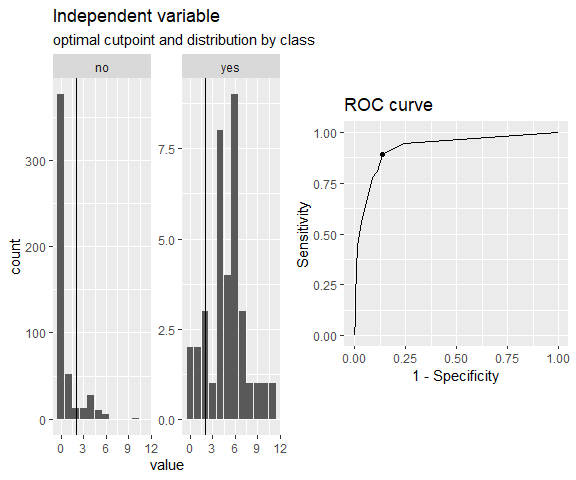When considering the optimality of a cutpoint, we can only make a judgement based on the sample at hand. Thus, the estimated cutpoint may not be optimal within the population or on unseen data, which is why we sometimes put the “optimal” in quotation marks.

cutpointr makes assumptions about the direction of the dependency between class and x, if direction and / or pos_class or neg_class are not specified. The same result as above can be achieved by manually defining direction and the positive / negative classes which is slightly faster, since the classes and direction don’t have to be determined:

opt_cut <- cutpointr(suicide, dsi, suicide, direction = ">=", pos_class = "yes",
neg_class = "no", method = maximize_metric, metric = youden)

opt_cut is a data frame that returns the input data and the ROC curve (and optionally the bootstrap results) in a nested tibble. Methods for summarizing and plotting the data and results are included (e.g. summary, plot, plot_roc, plot_metric)

To inspect the optimization, the function of metric values per cutpoint can be plotted using plot_metric, if an optimization function was used that returns a metric column in the roc_curve column. For example, the maximize_metric and minimize_metric functions do so:

plot_metric(opt_cut)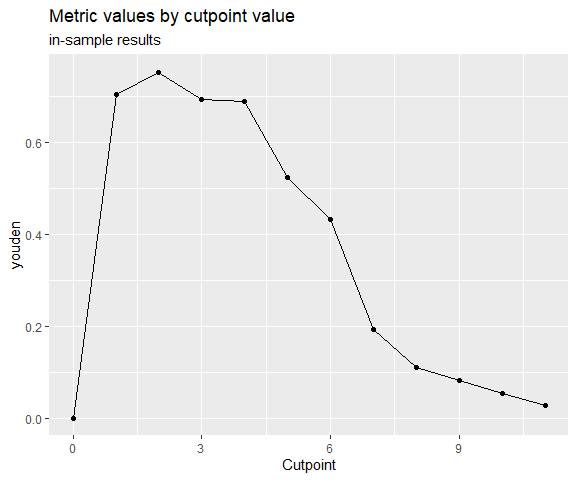Predictions for new data can be made using predict:

predict(opt_cut, newdata = data.frame(dsi = 0:5))
##  "no"  "no"  "yes" "yes" "yes" "yes"

## Features

• Calculation of optimal cutpoints in binary classification tasks
• Tidy output, integrates well with functions from the tidyverse
• Functions for plotting ROC curves, metric distributions and more
• Bootstrapping for simulating the cutpoint variability and for obtaining out-of-bag estimates of various metrics (as a form of internal validation) with optional parallelisation
• Multiple methods for calculating cutpoints
• Multiple metrics can be chosen for maximization / minimization
• Tidyeval

# Calculating cutpoints

## Method functions for cutpoint estimation

The included methods for calculating cutpoints are:

• maximize_metric: Maximize the metric function
• minimize_metric: Minimize the metric function
• maximize_loess_metric: Maximize the metric function after LOESS smoothing
• minimize_loess_metric: Minimize the metric function after LOESS smoothing
• maximize_spline_metric: Maximize the metric function after spline smoothing
• minimize_spline_metric: Minimize the metric function after spline smoothing
• maximize_gam_metric: Maximize the metric function after smoothing via Generalized Additive Models
• minimize_gam_metric: Minimize the metric function after smoothing via Generalized Additive Models
• maximize_boot_metric: Bootstrap the optimal cutpoint when maximizing a metric
• minimize_boot_metric: Bootstrap the optimal cutpoint when minimizing a metric
• oc_manual: Specify the cutoff value manually
• oc_mean: Use the sample mean as the “optimal” cutpoint
• oc_median: Use the sample median as the “optimal” cutpoint
• oc_youden_kernel: Maximize the Youden-Index after kernel smoothing the distributions of the two classes
• oc_youden_normal: Maximize the Youden-Index parametrically assuming normally distributed data in both classes

## Metric functions

The included metrics to be used with the minimization and maximization methods are:

• accuracy: Fraction correctly classified
• abs_d_sens_spec: The absolute difference of sensitivity and specificity
• abs_d_ppv_npv: The absolute difference between positive predictive value (PPV) and negative predictive value (NPV)
• roc01: Distance to the point (0,1) on ROC space
• cohens_kappa: Cohen’s Kappa
• sum_sens_spec: sensitivity + specificity
• sum_ppv_npv: The sum of positive predictive value (PPV) and negative predictive value (NPV)
• prod_sens_spec: sensitivity * specificity
• prod_ppv_npv: The product of positive predictive value (PPV) and negative predictive value (NPV)
• youden: Youden- or J-Index = sensitivity + specificity - 1
• odds_ratio: (Diagnostic) odds ratio
• risk_ratio: risk ratio (relative risk)
• p_chisquared: The p-value of a chi-squared test on the confusion matrix
• cost_misclassification: The sum of the misclassification cost of false positives and false negatives. Additional arguments: cost_fp, cost_fn
• total_utility: The total utility of true / false positives / negatives. Additional arguments: utility_tp, utility_tn, cost_fp, cost_fn
• F1_score: The F1-score (2 * TP) / (2 * TP + FP + FN)
• metric_constrain: Maximize a selected metric given a minimal value of another selected metric
• sens_constrain: Maximize sensitivity given a minimal value of specificity
• spec_constrain: Maximize specificity given a minimal value of sensitivity
• acc_constrain: Maximize accuracy given a minimal value of sensitivity

Furthermore, the following functions are included which can be used as metric functions but are more useful for plotting purposes, for example in plot_cutpointr, or for defining new metric functions: tp, fp, tn, fn, tpr, fpr, tnr, fnr, false_omission_rate, false_discovery_rate, ppv, npv, precision, recall, sensitivity, and specificity.

The inputs to the arguments method and metric are functions so that user-defined functions can easily be supplied instead of the built-in ones.

## Separate subgroups and bootstrapping

Cutpoints can be separately estimated on subgroups that are defined by a third variable, gender in this case. Additionally, if boot_runs is larger zero, cutpointr will carry out the usual cutpoint calculation on the full sample, just as before, and additionally on boot_runs bootstrap samples. This offers a way of gauging the out-of-sample performance of the cutpoint estimation method. If a subgroup is given, the bootstrapping is carried out separately for every subgroup which is also reflected in the plots and output.

set.seed(12)
opt_cut_b <- cutpointr(suicide, dsi, suicide, boot_runs = 1000)
opt_cut_b
## # A tibble: 1 x 16
##   direction optimal_cutpoint method          sum_sens_spec      acc sensitivity
##   <chr>                <dbl> <chr>                   <dbl>    <dbl>       <dbl>
## 1 >=                       2 maximize_metric       1.75179 0.864662    0.888889
##   specificity      AUC pos_class neg_class prevalence outcome predictor
##         <dbl>    <dbl> <fct>     <fct>          <dbl> <chr>   <chr>
## 1    0.862903 0.923779 yes       no         0.0676692 suicide dsi
##   data               roc_curve                 boot
##   <list>             <list>                    <list>
## 1 <tibble [532 x 2]> <roc_cutpointr [13 x 10]> <tibble [1,000 x 23]>

The returned object has the additional column boot which is a nested tibble that includes the cutpoints per bootstrap sample along with the metric calculated using the function in metric and various default metrics. The metrics are suffixed by _b to indicate in-bag results or _oob to indicate out-of-bag results:

opt_cut_b$boot ## [] ## # A tibble: 1,000 x 23 ## optimal_cutpoint AUC_b AUC_oob sum_sens_spec_b sum_sens_spec_o~ acc_b acc_oob ## <dbl> <dbl> <dbl> <dbl> <dbl> <dbl> <dbl> ## 1 2 0.957 0.884 1.80 1.71 0.874 0.842 ## 2 1 0.918 0.935 1.70 1.70 0.752 0.771 ## 3 2 0.920 0.946 1.79 1.73 0.874 0.864 ## 4 2 0.940 0.962 1.82 1.76 0.893 0.851 ## 5 2 0.849 0.96 1.66 1.76 0.848 0.887 ## 6 4 0.926 0.927 1.80 1.51 0.925 0.881 ## 7 2 0.927 0.919 1.74 1.78 0.885 0.862 ## 8 2 0.958 0.882 1.82 1.67 0.863 0.861 ## 9 4 0.911 0.923 1.80 1.53 0.914 0.879 ## 10 1 0.871 0.975 1.62 1.80 0.737 0.820 ## # ... with 990 more rows, and 16 more variables: sensitivity_b <dbl>, ## # sensitivity_oob <dbl>, specificity_b <dbl>, specificity_oob <dbl>, ## # cohens_kappa_b <dbl>, cohens_kappa_oob <dbl>, TP_b <dbl>, FP_b <dbl>, ## # TN_b <int>, FN_b <int>, TP_oob <dbl>, FP_oob <dbl>, TN_oob <int>, ## # FN_oob <int>, roc_curve_b <list>, roc_curve_oob <list> The summary and plots include additional elements that summarize or display the bootstrap results: summary(opt_cut_b) ## Method: maximize_metric ## Predictor: dsi ## Outcome: suicide ## Direction: >= ## Nr. of bootstraps: 1000 ## ## AUC n n_pos n_neg ## 0.9238 532 36 496 ## ## optimal_cutpoint sum_sens_spec acc sensitivity specificity tp fn fp tn ## 2 1.7518 0.8647 0.8889 0.8629 32 4 68 428 ## ## Predictor summary: ## Data Min. 5% 1st Qu. Median Mean 3rd Qu. 95% Max. SD NAs ## Overall 0 0.00 0 0 0.9210526 1 5.00 11 1.852714 0 ## no 0 0.00 0 0 0.6330645 0 4.00 10 1.412225 0 ## yes 0 0.75 4 5 4.8888889 6 9.25 11 2.549821 0 ## ## Bootstrap summary: ## Variable Min. 5% 1st Qu. Median Mean 3rd Qu. 95% Max. SD NAs ## optimal_cutpoint 1.00 1.00 2.00 2.00 2.12 2.00 4.00 4.00 0.72 0 ## AUC_b 0.83 0.88 0.91 0.93 0.92 0.94 0.96 0.98 0.02 0 ## AUC_oob 0.82 0.86 0.90 0.92 0.92 0.95 0.97 1.00 0.03 0 ## sum_sens_spec_b 1.57 1.67 1.72 1.76 1.76 1.80 1.84 1.89 0.05 0 ## sum_sens_spec_oob 1.37 1.56 1.66 1.72 1.71 1.78 1.86 1.90 0.09 0 ## acc_b 0.73 0.77 0.85 0.87 0.86 0.88 0.91 0.94 0.04 0 ## acc_oob 0.72 0.77 0.85 0.86 0.86 0.88 0.90 0.93 0.04 0 ## sensitivity_b 0.72 0.81 0.86 0.90 0.90 0.94 0.98 1.00 0.05 0 ## sensitivity_oob 0.44 0.67 0.80 0.87 0.86 0.93 1.00 1.00 0.10 0 ## specificity_b 0.72 0.76 0.85 0.86 0.86 0.88 0.91 0.94 0.04 0 ## specificity_oob 0.69 0.76 0.84 0.86 0.86 0.88 0.91 0.94 0.04 0 ## cohens_kappa_b 0.16 0.27 0.37 0.42 0.41 0.46 0.52 0.66 0.07 0 ## cohens_kappa_oob 0.15 0.25 0.34 0.39 0.39 0.44 0.51 0.62 0.08 0 plot(opt_cut_b)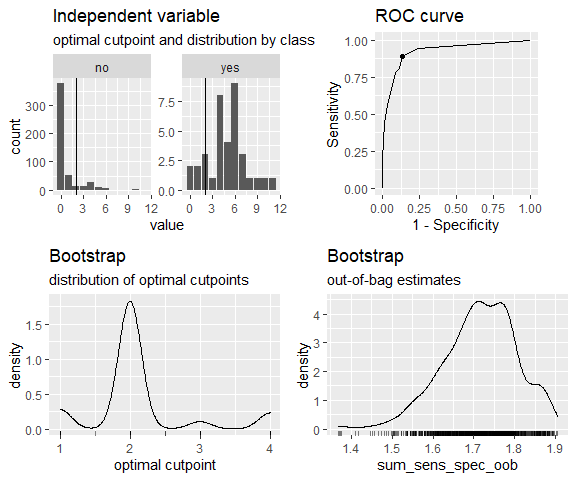### Parallelized bootstrapping Using foreach and doRNG the bootstrapping can be parallelized easily. The doRNG package is being used to make the bootstrap sampling reproducible. library(doParallel) cl <- makeCluster(2) # 2 cores registerDoParallel(cl) registerDoRNG(12) # Reproducible parallel loops using doRNG opt_cut <- cutpointr(suicide, dsi, suicide, gender, pos_class = "yes", direction = ">=", boot_runs = 1000, allowParallel = TRUE) stopCluster(cl) opt_cut # Additional features ## Finding all cutpoints with acceptable performance By default, most packages only return the “best” cutpoint and disregard other cutpoints with quite similar performance, even if the performance differences are minuscule. cutpointr makes this process more explicit via the tol_metric argument. For example, if all cutpoints are of interest that achieve at least an accuracy within 0.05 of the optimally achievable accuracy, tol_metric can be set to 0.05 and also those cutpoints will be returned. In the case of the suicide data and when maximizing the sum of sensitivity and specificity, empirically the cutpoints 2 and 3 lead to quite similar performances. If tol_metric is set to 0.05, both will be returned. library(tidyr) library(dplyr) ## ## Attaching package: 'dplyr' ## The following objects are masked from 'package:stats': ## ## filter, lag ## The following objects are masked from 'package:base': ## ## intersect, setdiff, setequal, union opt_cut <- cutpointr(suicide, dsi, suicide, metric = sum_sens_spec, tol_metric = 0.05, break_ties = c) ## Assuming the positive class is yes ## Assuming the positive class has higher x values ## Multiple optimal cutpoints found, applying break_ties. opt_cut |> select(optimal_cutpoint, sum_sens_spec) |> unnest(cols = c(optimal_cutpoint, sum_sens_spec)) ## # A tibble: 2 x 2 ## optimal_cutpoint sum_sens_spec ## <dbl> <dbl> ## 1 2 1.75 ## 2 1 1.70 ## Manual and mean / median cutpoints Using the oc_manual function the optimal cutpoint will not be determined based on, for example, a metric but is instead set manually using the cutpoint argument. This is useful for supplying and evaluating cutpoints that were found in the literature or in other external sources. The oc_manual function could also be used to set the cutpoint to the sample mean using cutpoint = mean(data$x). However, this may introduce bias into the bootstrap validation procedure, since the actual mean of the population is not known and thus the mean to be used as the cutpoint should be automatically determined in every resample. To do so, the oc_mean and oc_median functions can be used.

set.seed(100)
opt_cut_manual <- cutpointr(suicide, dsi, suicide, method = oc_manual,
cutpoint = mean(suicide\$dsi), boot_runs = 1000)
set.seed(100)
opt_cut_mean <- cutpointr(suicide, dsi, suicide, method = oc_mean, boot_runs = 1000)

## Nonstandard evaluation via tidyeval

The arguments to cutpointr do not need to be enclosed in quotes. This is possible thanks to nonstandard evaluation of the arguments, which are evaluated on data.

Functions that use nonstandard evaluation are often not suitable for programming with. The use of nonstandard evaluation may lead to scoping problems and subsequent obvious as well as possibly subtle errors. cutpointr uses tidyeval internally and accordingly the same rules as for programming with dplyr apply. Arguments can be unquoted with !!:

myvar <- "dsi"
cutpointr(suicide, !!myvar, suicide)

## Midpoints

So far - which is the default in cutpointr - we have considered all unique values of the predictor as possible cutpoints. An alternative could be to use a sequence of equidistant values instead, for example in the case of the suicide data all integers in $$[0, 10]$$. However, with very sparse data and small intervals between the candidate cutpoints (i.e. a ‘dense’ sequence like seq(0, 10, by = 0.01)) this leads to the uninformative evaluation of large ranges of cutpoints that all result in the same metric value. A more elegant alternative, not only for the case of sparse data, that is supported by cutpointr is the use of a mean value of the optimal cutpoint and the next highest (if direction = ">=") or the next lowest (if direction = "<=") predictor value in the data. The result is an optimal cutpoint that is equal to the cutpoint that would be obtained using an infinitely dense sequence of candidate cutpoints and is thus usually more efficient computationally.

This behavior can be activated by setting use_midpoints = TRUE, which is the default. If we use this setting, we obtain an optimal cutpoint of 1.5 for the complete sample on the suicide data instead of 2 when maximizing the sum of sensitivity and specificity.

Assume the following small data set:

dat <- data.frame(outcome = c("neg", "neg", "neg", "pos", "pos", "pos", "pos"),
pred    = c(1, 2, 3, 8, 11, 11, 12))

Since the distance of the optimal cutpoint (8) to the next lowest observation (3) is rather large we arrive at a range of possible cutpoints that all maximize the metric. In the case of this kind of sparseness it might for example be desirable to classify a new observation with a predictor value of 4 as belonging to the negative class. If use_midpoints is set to TRUE, the mean of the optimal cutpoint and the next lowest observation is returned as the optimal cutpoint, if direction is >=. The mean of the optimal cutpoint and the next highest observation is returned as the optimal cutpoint, if direction = "<=".

opt_cut <- cutpointr(dat, x = pred, class = outcome, use_midpoints = TRUE)
## Assuming the positive class is pos
## Assuming the positive class has higher x values
plot_x(opt_cut)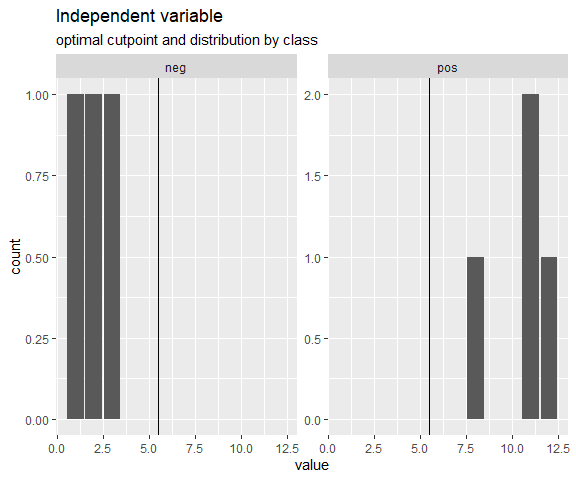A simulation demonstrates more clearly that setting use_midpoints = TRUE avoids biasing the cutpoints. To simulate the bias of the metric functions, the predictor values of both classes were drawn from normal distributions with constant standard deviations of 10, a constant mean of the negative class of 100 and higher mean values of the positive class that are selected in such a way that optimal Youden-Index values of 0.2, 0.4, 0.6, and 0.8 result in the population. Samples of 9 different sizes were drawn and the cutpoints that maximize the Youden-Index were estimated. The simulation was repeated 10000 times. As can be seen by the mean error, use_midpoints = TRUE eliminates the bias that is introduced by otherwise selecting the value of an observation as the optimal cutpoint. If direction = ">=", as in this case, the observation that represents the optimal cutpoint is the highest possible cutpoint that leads to the optimal metric value and thus the biases are positive. The methods oc_youden_normal and oc_youden_kernel are always unbiased, as they don’t select a cutpoint based on the ROC-curve or the function of metric values per cutpoint.